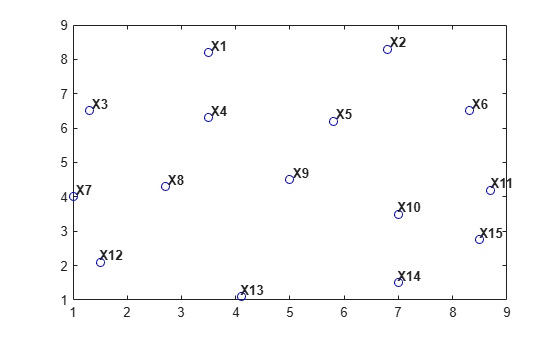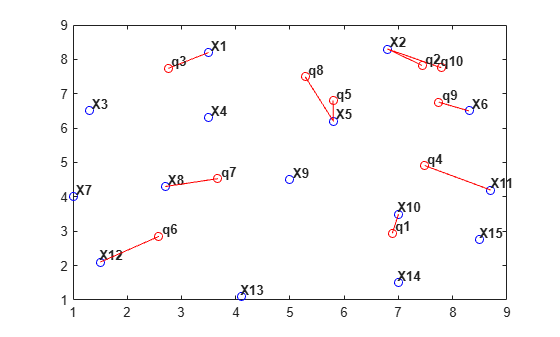# Spatial Searching

### Introduction

MATLAB® provides the necessary functions for performing a spatial search using either a Delaunay triangulation or a general triangulation. The search queries that MATLAB supports are:

• Nearest-neighbor search (sometimes called closest-point search or proximity search).

• Point-location search (sometimes called point-in-triangle search or point-in-simplex search, where a simplex is a triangle, tetrahedron or higher dimensional equivalent).

Given a set of points `X` and a query point `q` in Euclidean space, the nearest-neighbor search locates a point `p` in `X` that is closer to `q` than to any other point in `X`. Given a triangulation of `X`, the point-location search locates the triangle or tetrahedron that contains the query point `q`. Since these methods work for both Delaunay as well as general triangulations, you can use them even if a modification of the points violates the Delaunay criterion. You also can search a general triangulation represented in matrix format.

While MATLAB supports these search schemes in `N` dimensions, exact spatial searches usually become prohibitive as the number of dimensions extends beyond 3-D. You should consider approximate alternatives for large problems in up to 10 dimensions.

### Nearest-Neighbor Search

There are a few ways to compute nearest-neighbors in MATLAB, depending on the dimensionality of the problem:

This example shows how to perform a nearest-neighbor search in 2-D with `delaunayTriangulation`.

Begin by creating a random set of 15 points.

```X = [3.5 8.2; 6.8 8.3; 1.3 6.5; 3.5 6.3; 5.8 6.2; 8.3 6.5;... 1 4; 2.7 4.3; 5 4.5; 7 3.5; 8.7 4.2; 1.5 2.1; 4.1 1.1; ... 7 1.5; 8.5 2.75];```

Plot the points and add annotations to show the ID labels.

```plot(X(:,1),X(:,2),'ob') hold on vxlabels = arrayfun(@(n) {sprintf('X%d', n)}, (1:15)'); Hpl = text(X(:,1)+0.2, X(:,2)+0.2, vxlabels, 'FontWeight', ... 'bold', 'HorizontalAlignment','center', 'BackgroundColor', ... 'none'); hold off```

Create a Delaunay triangulation from the points.

`dt = delaunayTriangulation(X);`

Create some query points and for each query point find the index of its corresponding nearest neighbor in `X` using the `nearestNeighbor` method.

```numq = 10; rng(0,'twister'); q = 2+rand(numq,2)*6; xi = nearestNeighbor(dt, q);```

Add the query points to the plot and add line segments joining the query points to their nearest neighbors.

```xnn = X(xi,:); hold on plot(q(:,1),q(:,2),'or'); plot([xnn(:,1) q(:,1)]',[xnn(:,2) q(:,2)]','-r'); vxlabels = arrayfun(@(n) {sprintf('q%d', n)}, (1:numq)'); Hpl = text(q(:,1)+0.2, q(:,2)+0.2, vxlabels, 'FontWeight', ... 'bold', 'HorizontalAlignment','center', ... 'BackgroundColor','none'); hold off```Performing a nearest-neighbor search in 3-D is a direct extension of the 2-D example based on `delaunayTriangulation`.

For 4-D and higher, use the `delaunayn` and `dsearchn` functions as illustrated in the following example:

Create a random sample of points in 4-D and triangulate the points using `delaunayn`:

```X = 20*rand(50,4) -10; tri = delaunayn(X);```

Create some query points and for each query point find the index of its corresponding nearest-neighbor in `X` using the `dsearchn` function:

```q = rand(5,4); xi = dsearchn(X,tri, q);```

The `nearestNeighbor` method and the `dsearchn` function allow the Euclidean distance between the query point and its nearest-neighbor to be returned as an optional argument. In the 4-D example, you can compute the distances, `dnn`, as follows:

`[xi,dnn] = dsearchn(X,tri,q);`

### Point-Location Search

A point-location search is a triangulation search algorithm that locates the simplex (triangle, tetrahedron, and so on) enclosing a query point. As in the case of the nearest-neighbor search, there are a few approaches to performing a point-location search in MATLAB, depending on the dimensionality of the problem:

• For 2-D and 3-D, use the class-based approach with the `pointLocation` method provided by the `triangulation` class and inherited by the `delaunayTriangulation` class.

• For 4-D and higher, use the `delaunayn` function to construct the triangulation and the complementary `tsearchn` function to perform the point-location search. Although supporting 2-D and 3-D, these N-D functions are not as general and efficient as the triangulation search methods.

This example shows how to use the `delaunayTriangulation` class to perform a point location search in 2-D.

Begin with a set of 2-D points.

```X = [3.5 8.2; 6.8 8.3; 1.3 6.5; 3.5 6.3; 5.8 6.2; ... 8.3 6.5; 1 4; 2.7 4.3; 5 4.5; 7 3.5; 8.7 4.2; ... 1.5 2.1; 4.1 1.1; 7 1.5; 8.5 2.75];```

Create the triangulation and plot it showing the triangle ID labels at the incenters of the triangles.

```dt = delaunayTriangulation(X); triplot(dt); hold on ic = incenter(dt); numtri = size(dt,1); trilabels = arrayfun(@(x) {sprintf('T%d', x)}, (1:numtri)'); Htl = text(ic(:,1), ic(:,2), trilabels, 'FontWeight', ... 'bold', 'HorizontalAlignment', 'center', 'Color', ... 'blue'); hold off```

Now create some query points and add them to the plot. Then find the index of the corresponding enclosing triangles using the `pointLocation` method.

```q = [5.9344 6.2363; 2.2143 2.1910; 7.0948 3.6615; 7.6040 2.2770; 6.0724 2.5828; 6.5464 6.9407; 6.4588 6.1690; 4.3534 3.9026; 5.9329 7.7013; 3.0271 2.2067]; hold on; plot(q(:,1),q(:,2),'*r'); vxlabels = arrayfun(@(n) {sprintf('q%d', n)}, (1:10)'); Hpl = text(q(:,1)+0.2, q(:,2)+0.2, vxlabels, 'FontWeight', ... 'bold', 'HorizontalAlignment','center', ... 'BackgroundColor', 'none'); hold off````ti = pointLocation(dt,q);`

Performing a point-location search in 3-D is a direct extension of performing a point-location search in 2-D with `delaunayTriangulation`.

For 4-D and higher, use the `delaunayn` and `tsearchn` functions as illustrated in the following example:

Create a random sample of points in 4-D and triangulate them using `delaunayn`:

```X = 20*rand(50,4) -10; tri = delaunayn(X);```

Create some query points and find the index of the corresponding enclosing simplices using the tsearchn function:

```q = rand(5,4); ti = tsearchn(X,tri,q);```

The `pointLocation` method and the `tsearchn` function allow the corresponding barycentric coordinates to be returned as an optional argument. In the 4-D example, you can compute the barycentric coordinates as follows:

`[ti,bc] = tsearchn(X,tri,q);`

The barycentric coordinates are useful for performing linear interpolation. These coordinates provide you with weights that you can use to scale the values at each vertex of the enclosing simplex. See Interpolating Scattered Data for further details.